# Digital Control

Publish Date: Nov 29, 2010 | 7 Ratings | 2.57 out of 5 | Print | Submit your review

## Overview

The purpose of this tutorial is to show how to use LabVIEW and the LabVIEW Control Design and Simulation Module to work with discrete functions either in transfer function or state-space form to design digital control systems.

These tutorials are based on the Control Tutorials developed by Professor Dawn Tilbury of the Mechanical Engineering department at the University of Michigan and Professor Bill Messner of the Department of Mechanical Engineering at Carnegie Mellon University and were developed with their permission.

### 1. Introduction

The figure below shows the typical continuous feedback system that we have been considering so far in this tutorial. Almost all of the continuous controllers can be built using analog electronics.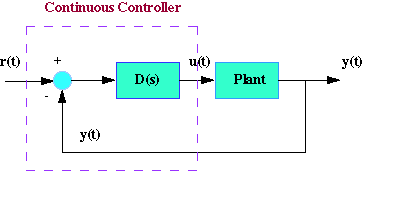Figure 1: Continuous Feedback System

The continuous controller, enclosed in the dashed square, can be replaced by a digital controller, shown below in Figure 2, that performs same control task as the continuous controller. The basic difference between these controllers is that the digital system operates on discrete signals (or samples of the sensed signal) rather than on continuous signals.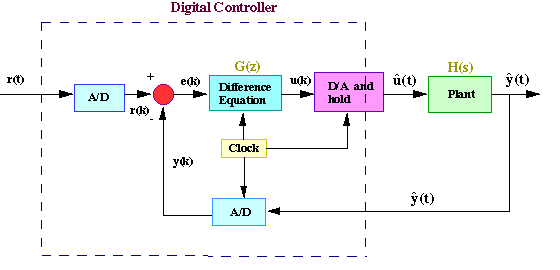Figure 2: Discrete Feedback System

Different types of signals in the above digital schematic can be represented by the following plots.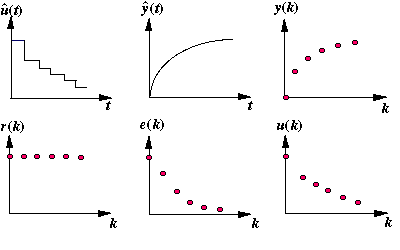Figure 3: Continuous and Discrete Signals

The purpose of this Digital Control Tutorial is to show you how to use LabVIEW to work with discrete functions either in transfer function or state-space form to design digital control systems.

### 2. Zero-order Hold Equivalence

In the above schematic of the digital control system, we see that the digital control system contains both discrete and the continuous portions. When designing a digital control system, we need to find the discrete equivalent of the continuous portion so that we only need to deal with discrete functions.

For this technique, we will consider the following portion of the digital control system and rearrange as follows.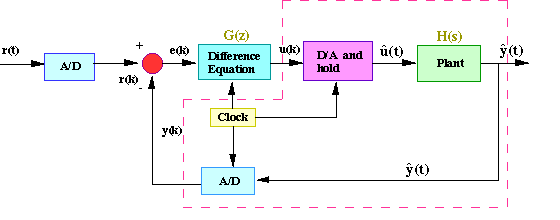Figure 4: Discrete Feedback System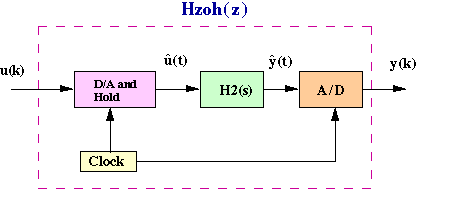Figure 5: Discrete Feedback System Rearranged

The clock connected to the D/A and A/D converters supplies a pulse every T seconds, and each D/A and A/D converter sends a signal only when the pulse arrives. The purpose of having this pulse is to require that Hzoh(z) have only samples u(k) to work on and produce only samples of output y(k); thus, Hzoh(z) can be realized as a discrete function.

The philosophy of the design is the following. We want to find a discrete function Hzoh(z) so that for a piecewise constant input to the continuous system H(s), the sampled output of the continuous system equals the discrete output. Suppose the signal u(k) represents a sample of the input signal. There are techniques for taking this sample u(k) and holding it to produce a continuous signal uhat(t). The sketch in Figure 6 below shows that the uhat(t) is held constant at u(k) over the interval kT to (k+1)T. This operation of holding uhat(t) constant over the sampling time is called zero-order hold.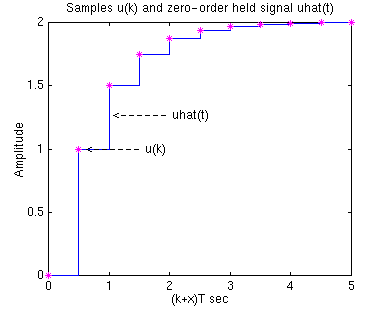Figure 6: Zero-order Hold

The zero-order held signal uhat(t) goes through H2(s) and A/D to produce the output y(k) that will be the piecewise same signal as if the discrete signal u(k) goes through Hzoh(z) to produce the discrete output y(k).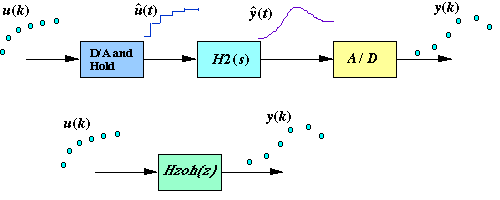Figure 7: Output Signal

Now we will redraw the schematic, placing Hzoh(z) in place of the continuous portion.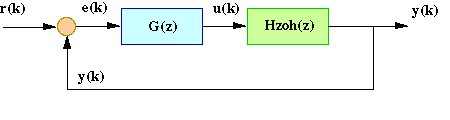Figure 8: Redrawn Schematic

By placing Hzoh(z), we can design digital control systems dealing with only discrete functions.

There are certain cases where the discrete response does not match the continuous response due to a hold circuit implemented in digital control systems. In these cases, the hold causes a lagging effect in the discrete response. This effect can be reduced by decreasing the sampling time.

### LabVIEW Graphical Approach

To convert continuous systems to discrete systems, you can use the CD Convert Continuous to Discrete VI from the Model Conversion section of the Control Design palette.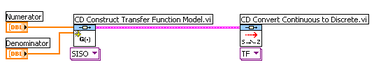Figure 9: Convert Continuous Model to Discrete Model

### LabVIEW MathScript Approach

Alternatively, the MathScript function called c2d also converts a given continuous system to a discrete system. For example: sys_d = c2d(sys,Ts,'zoh').

### Result

Both of these methods use the zero-order hold operation explained above.

### 4. Stability and Transient Response

For continuous systems, we know that certain behaviors result from different pole locations in the s-plane. For instance, a system is unstable when any pole is located to the right of the imaginary axis. For discrete systems, we can analyze the system behaviors from different pole locations in the z-plane. The characteristics in the z-plane can be related to those in the s-plane by the equation: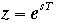In this equation, T = sampling time (sec/sample), s = location in the s-plane, and z = location in the z-plane.

Figure 10 below shows the mapping of lines of constant damping ratio (zeta) and natural frequency (Wn) from the s-plane to the z-plane using the expression shown above.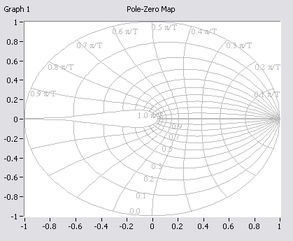Figure 10: Z-Plane

If you noticed in the z-plane, the stability boundary is no longer the imaginary axis, but is now the unit circle |z|=1. The system is stable when all poles are located inside the unit circle and unstable when any pole is located outside.

For analyzing the transient response from pole locations in the z-plane, the following three equations used in continuous system designs are applicable: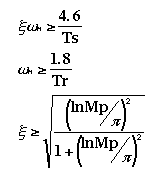In the above equations, zeta = Damping ratio, Wn = Natural frequency (rad/sec), Ts = Settling time, Tr = Rise time, and Mp = Maximum overshoot.

Important: The natural frequency (Wn) in the z-plane has the unit of rad/sample, but when you use the equations shown above, the Wn must be in the unit of rad/sec.

### 5. Plotting Poles and Zeros of a Discrete System

Suppose we have the following discrete transfer function: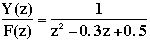### LabVIEW Graphical Approach

We can plot the zeros and poles of this function using the CD Pole-Zero Map VI.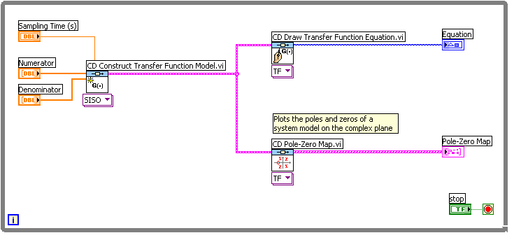Figure 11: Plotting Poles and Zeros (Download )

### LabVIEW MathScript Approach

Alternatively, we can use MathScript to plot the poles and zeros. Open the MathScript Window and enter the following commands. Running this m-file in the command window gives you a plot of poles and zeros, with the lines of constant damping ratio and natural frequency.

numDz = 1;

denDz = [1 -0.3 0.5];

sys = tf(numDz,denDz,1/20)

pzmap(sys)

axis([-1 1 -1 1])

zgrid on

### Result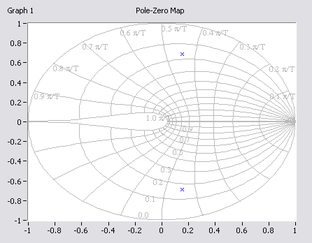Figure 12: Pole-Zero Map

From this plot, we see that poles are located approximately at the natural frequency of 9pi/20T (rad/sample) and the damping ratio of 0.25. Assuming that we have a sampling time of 1/20 sec (which leads to Wn = 28.2 rad/sec) and using three equations shown above, we can determine that this system should have the rise time of 0.06 sec, a settling time of 0.65 sec and a maximum overshoot of 45% (0.45 more than the steady-state value).

Let's obtain the step response and see if these are correct.

### LabVIEW Graphical Approach

Add the CD Step Response VI to your block diagram.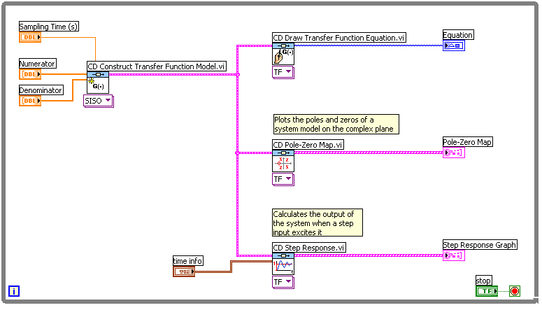Figure 13: Pole-Zero Map with Step Response (Download )

### LabVIEW MathScript Approach

If you are using the MathScript Window, add the following commands to the command window.

sys = tf(numDz,denDz,1/20);

step(sys,2.5);

### Result

You should get the following step response: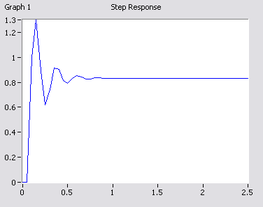Figure 14: Step Response

As you can see from the plot, the rise time, settling time and overshoot came out to be what we expected. This shows how you can use the locations of poles and the above three equations to analyze the transient response of the system.

### 6. Discrete Root-Locus

The root-locus is the locus of points where roots of characteristic equation can be found as a single gain is varied from zero to infinity. The characteristic equation of a unity feedback system is: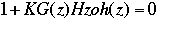In this equation, G(z) is the compensator implemented in the digital controller, and Hzoh(z) is the plant transfer function.

The mechanics of drawing the root-loci are exactly the same in the z-plane as in the s-plane. Recall from the Root Locus Tutorial, we used the MathScript function called sgrid on to turn on root-locus grid lines, in order to find the root-locus region that gives an acceptable gain (K). For the discrete root-locus analysis, we will use the function zgrid on. The command zgrid on draws lines of constant damping ratio (zeta) and natural frequency (Wn).

Suppose we have the following discrete transfer function: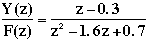The requirements are a damping ratio greater than 0.6 and a natural frequency greater than 0.4 rad/sample (these can be found from design requirements, sampling time (sec/sample) and three equations shown in the previous section).

### LabVIEW MathScript Approach

In the MathScript Window, enter the following commands. Running this should give you a root-locus plot.

numDz = [1 -0.3];

denDz = [1 -1.6 0.7];

sys = tf(numDz,denDz,1);

rlocus(sys)

axis([-1 1 -1 1])

zgrid on

### Result

The following figure shows the plot that you should now see. The red and green lines have been superimposed on the plot.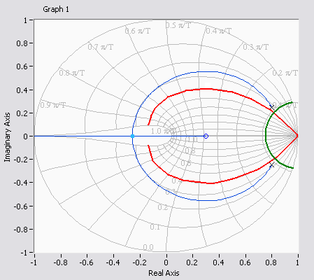Figure 15: Discrete Root-Locus

From the plot in Figure 15, you should realize that the system is stable because all poles are located inside the unit circle. In the figure, the red line superimposed on the graph indicates pole locations with a damping ratio (Zeta) of 0.4. Similarly, the green line shows locations with a natural frequency (Wn) of 0.3. The natural frequency is greater than 0.3 outside the constant-Wn line, and the damping ratio is greater than 0.4 inside the constant-zeta line. In this example, we do have the root-locus drawn in the desired region. Therefore, a gain (K) chosen from one of the loci in the desired region should give you the response that satisfies design requirements.